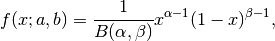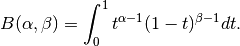# numpy.random.beta¶

numpy.random.beta(a, b, size=None)

The Beta distribution over [0, 1].

The Beta distribution is a special case of the Dirichlet distribution, and is related to the Gamma distribution. It has the probability distribution functionwhere the normalisation, B, is the beta function,It is often seen in Bayesian inference and order statistics.

Parameters : a : float Alpha, non-negative. b : float Beta, non-negative. size : tuple of ints, optional The number of samples to draw. The ouput is packed according to the size given. out : ndarray Array of the given shape, containing values drawn from a Beta distribution.

#### Previous topic

numpy.random.permutation

#### Next topic

numpy.random.binomial